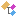﻿ Nevron.Nov.Diagram.Expressions Namespace
Nevron Open Vision Documentation
Inheritance Hierarchy
In This Topic
Nevron.Nov.Diagram.Expressions Namespace
In This Topic
Classes
ClassDescriptionAn expression that is applied to the X and Y properties of a shape control point. This type of expression works in collaboration with the Glue object of the control point.An expression that is applied to the BeginX, BeginY, EndX and EndY properties of a shape. This type of expression works in collaboration with the BeginGlue/EndGlue objects of the shape.NCompositeHeightFactorFxNGroupWidthFactorFx.An expression that is applied to the PinX, PinY and Angle properties of a text block. This type of expression works in collaboration with the ImageBlockGlue object of the text block.An expression that is applied to the PinX, PinY and Angle properties of a shape. This type of expression works in collaboration with the MasterGlue object of the shape.An expression that is applied to the X, Y, DirX and DirY properties of a shape port. This type of expression works in collaboration with the Glue object of the port.NResizeInGroupFx.An expression that is applied to the PinX, PinY and Angle properties of a shape block. Serves as base class for NImageBlockGlueFx and NTextBlockGlueFx.An expression that calculates the angle that the line connecting the Begin and End points of a shape forms with the X axis (e.g. =ATAN2(EndY-BeginY,EndX-BeginX)). This expression is commonly used as Angle of 1D shapes.An expression that calculates the difference between the the shape BeginX and EndX values. This expression is commonly used as Width of 1DShapes.An expression that calculates the difference between the the shape EndY and BeginY values. This expression is commonly used as Width of 1DShapes.An expression that calculates the length of the line connecting the Begin and End points of a shape (e.g. =SQRT((EndX-BeginX)^2+(EndY-BeginY)^2)). This expression is commonly used as Width of 1DShapes.An expression that calculates middle X coordinate of the line connecting the Begin and End points of a shape (e.g. (BeginX + EndX) / 2). This expression is commonly used as PinX location of 1DShapes.An expression that calculates middle Y coordinate of the line connecting the Begin and End points of a shape (e.g. (BeginY + EndY) / 2). This expression is commonly used as PinY location of 1DShapes.NShapeHeightFactorExpressionNShapeWidthFactorExpressionAn expression that is applied to the PinX, PinY and Angle properties of a text block. This type of expression works in collaboration with the TextBlockGlue object of the text block.